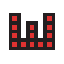#Wiki

Reso-nance numérique | Arts et cultures libres

### Panneau latéral

Catégories

Liens

logiciels:terminologie-3d:accueil

# Terminologie 3D

## 2D et 3D PRIMITIVES

Cercle, carré, rectangle, sphère, cylindre, tore, cone, …

## OPÉRATIONS BOOLÉENNES

Union, différence, intersection

## TRANSFORMATIONS GÉOMÉTRIQUES

translation, rotation, scale (homothétie), mirroir, …

## VERTEX = SOMMET

“The basic object used in mesh modelling is a vertex, a point in three dimensional space.“

“In geometry, a vertex (plural vertices) is a special kind of point which describes the corners or intersections of geometric shapes.Vertices are commonly used in computer graphics to define the corners of surfaces (typically triangles) in 3D models, where each such point is given as a vector. A vertex of a polygon is the point of intersection of two edges, a vertex of a polyhedron is the point of intersection of three or more edges or face.” Vertex geometry, Wikipedia, retrieved 17:25, 23 March 2010 (UTC)

## EDGE = ARÊTE

Two vertices connected by a straight line become an edge.

## POLYGON

Three vertices, connected to the each other by three edges, define a triangle, which is the simplest polygon (face) in Euclidean space. More complex polygons can be created out of multiple triangles, or as a single object with more than 3 vertices. Four sided polygons (generally referred to as quads) and triangles are the most common shapes used in polygonal modeling.

## FACES

The faces or facets define the surface of a 3-dimensional object, i.e. what is between “outside” and “inside”.

## ELEMENT

A group of polygons, connected to each other by shared vertices, is generally referred to as an element. Each of the polygons making up an element is called a face.” (Polygonal modeling, Wikipedia

## MESH = MAILLAGE

“A group of polygons which are connected together by shared vertices is referred to as a mesh. In order for a mesh to appear attractive when rendered, it is desirable that it be non-self-intersecting, meaning that no edge passes through a polygon. Another way of looking at this is that the mesh cannot pierce itself. It is also desirable that the mesh not contain any errors such as doubled vertices, edges, or faces. For some purposes it is important that the mesh be a manifold – that is, that it does not contain holes or singularities (locations where two distinct sections of the mesh are connected by a single vertex).” Vertex geometry, Wikipedia, retrieved 17:25, 23 March 2010 (UTC)

## NORMAL

The normal or surface normal is a vector that is perpendicular to a face. Surface normal, Wikipedia, retrieved 15:07, 20 November 2011 (UTC)

In many formats, e.g. STL and OpenSCAD, the following principle applies: “(i) The normal and each vertex of every facet are specified by three coordinates each, so there is a total of 12 numbers stored for each facet. (ii) Each facet is part of the boundary between the interior and the exterior of the object. The orientation of the facets (which way is ``out and which way is ``in) is specified redundantly in two ways which must be consistent. First, the direction of the normal is outward. Second, the vertices are listed in counterclockwise order when looking at the object from the outside (right-hand rule). (iii) Each triangle must share two vertices with each of its adjacent triangles. This is known as vertex-to-vertex rule. (iv) The object represented must be located in the all-positive octant (all vertex coordinates must be positive). ” (Daniel Rypl, Zdenek Bittnar, 2004, retrieved April 2010.

The normal or surface normal is a vector that is perpendicular to a face. Surface normal, Wikipedia, retrieved 15:07, 20 November 2011 (UTC)

/home/resonancg/www/wiki/data/pages/logiciels/terminologie-3d/accueil.txt · Dernière modification: 2018/01/22 11:06 de resonance

### Outils de la page